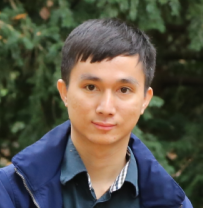Thông tin chungTS. Võ Ngọc Thiệu Tiến sĩ 2016 Giảng viên Email: vongocthieu@tdtu.edu.vn

A. Quá trình đào tạo và hoạt động khoa học

1. Quá trình đào tạo

• 2013 – 2016: Tiến sĩ Toán, Research Institute of Symbolic Computation, Johannes Kepler University, Linz, Austria
• 2011 – 2013: Thạc sĩ Toán, University of Padua, Italy và University of Bordeaux, France.
• 2006 – 2011: Cử nhân Toán và Tin học, Đại học Sư Phạm TPHCM, Vit Nam.

2. Quá trình hoạt động chuyên môn

• 2017– nay: Viên chức giảng dạy tại Trường Đại học Tôn Đức Thắng.

3. Lĩnh vực nghiên cứu

Algebraic theory for algebraic differential equations

Numerical methods for problems in fractional calculus

Computer algebra and its applications in Commutative algebra and Lie algebra

Mathematics foundations for deep learning

4. Hướng dẫn luận án

Đã hướng dẫn 10 ThS và 04 Cử nhân. Sau đây là 10 ThS:

1. Cô Thị Ngọc Linh (07/2018)
2. Trần Thị Bích Ngọc (11/2019)
3. Phan Thị Thanh Cảnh (11/2019)
4. Siu H'Liên (11/2019)
5. Vũ Thị Liên Hương (9/2020)
6. Phan Đức Thịnh (3/2021)
7. Nguyễn Đình Nghĩa (3/2021)
8. Đỗ Thị  Nhàn (9/2021)
9. Dư Hương Mỹ Linh
10. Trần Thị Thu Thảo

5. Mời đi hợp tác khoa học

1. Mathematics Department, Graduate Center, City University of New York, New York, US (3/2016-8/2016).

B. Công bố Khoa học

1. Bài báo trên tạp chí khoa học

 H.T.B. Ngo, M. Razzaghi, T.N. Vo. Fractional-order Chelyshkov wavelet method for solving variable-order fractional differential equations and an application in variable-order fractional relaxation system, Numerical Algorithms. Accepted.

 V.A. Le, H.Q. Duong, T.A. Nguyen, H.T. T. Cao, T.N. Vo. On the problem of classifying solvable Lie algebras having small codimensional derived algebras, Communications in Algebra. 2022.

 S. Falkensteiner, Y. Zhang, T.N. Vo. On Existence and Uniqueness of Formal Power Series Solutions of Algebraic Ordinary Differential Equations. Mediterranean Journal of Mathematics, 19(2022)74.

 M. Razzaghi, T.N. Vo. Numerical solutions for distributed-order fractional optimal control problems by using Müntz-Legendre wavelets. Proceeding of the Royal Society A, 478(2022)2258.

 L.T.N. Giau, P.T. Toan, T.N. Vo, Dedekind-Mertens lemma for power series in an arbitrary set of indeterminates, Vietnam Journal of Mathematics, 50(2022)45-58.

 T.N. Vo, M. Razzaghi, P.T. Toan. Fractional-order generalized Taylor wavelet method for systems of nonlinear fractional differential equations with application to human respiratory syncytial virus infection, Soft Computing, 26(2022)165-173.

 B. Yuttanan, M. Razzaghi, T.N. Vo. Legendre wavelet method for fractional delay differential equations. Applied Numerical Mathematics 168(2021)127-142.

 B. Yuttanan, M. Razzaghi, T.N. Vo. Fractional-order generalized Legendre wavelets and their applications to fractional Riccati differential equations. International Journal of Nonlinear Sciences and Numerical Simulation, 2021. Online First.

 A. Ovchinnikov, G. Pogudin, T. N. Vo. Bounds for elimination of unknowns in systems of differential-algebraic equations. International Mathematics Research Notices, 2021. Online First.

 T.N. Vo, M. Razzaghi, P.T. Toan. A numerical method for solving variable-order fractional diffusion equations using fractional-order Taylor wavelets, Numerical Methods for Partial Differential Equations, 37(2021)2668-2686.

 B. Yuttanan, M. Razzaghi, T.N. Vo. A numerical method based on fractional-order generalized Taylor wavelets for solving distributed-order fractional partial differential equations. Applied Numerical Mathematics, 160(2021)349-367.

 B. Yuttanan, M. Razzaghi, T.N. Vo. A fractional-order generalized Taylor wavelet method for nonlinear fractional delay and nonlinear fractional pantograph differential equations, Mathematical Methods in the Applied Sciences, 44(2021)4156-4175.

 P. T. Toan, T.N. Vo, M. Razzaghi. Taylor wavelet method for fractional delay differential equations, Engineering with Computers, 37(2021)231-240.

 H.T.B. Ngo, T.N. Vo, M. Razzaghi. An Effective Method for Solving Nonlinear Fractional Differential Equations, Engineering with Computers, 2020. Online First.

 T. N. Vo, Y. Zhang. Rational Solutions of High-Order Algebraic Ordinary Differential Equations, Journal of Systems Science and Complexity 33(2020)821-835.

 T. N. Vo, Y. Zhang. Rational solutions of first-order algebraic difference equations. Advances in Applied Mathematics. 117(2020)102018.

 V. A. Le, T. A. Nguyen, T. T. C. Nguyen, T. T. M. Nguyen, T. N. Vo. Applying matrix theory to classify real solvable Lie algebras having 2-dimensional derived ideals, Linear Algebra and its Applications 588(2020)282-303.

 P. Vichitkunakorn, T. N. Vo, M. Razzaghi. A numerical method for fractional pantograph differential equations based on Taylor wavelets, Transactions of the Institute of Measurement and Control,  42(2020)1334-1344.

 T. N. Vo, P. T. Toan. The power series Dedekind-Mertens number, Communications in Algebra 47(2019):3481-3489.

 E. Amzallag, G. Pogudin, M. Sun, T. N. Vo. Complexity of Triangular Representations of Algebraic Sets, Journal of Algebra, 523(2019)342-364.

 T. N. Vo, G. Grasegger, F. Winkler. Computation of all rational solutions of first-order algebraic ODEs, Advances in Applied Mathematics 98(2018)1-24.

 T. N. Vo, G. Grasegger, F. Winkler. Deciding the existence of rational general solutions for first-order algebraic ODEs, Journal of Symbolic Computation 87(2018)127-139.

2. Bài báo trong kỷ yếu hội nghị quốc tế

 T. N. Vo, An algebraic method for quasi-linear first-order ODEs, In Proceedings of the International Conference on Mathematics: Recent Advances in Algebra, Numerical Analysis, Applied Analysis and Statistics, ITM Web of Conferences, 20(2018)01006.

 G. Grasegger, T. N. Vo, An algebraic-geometric method for computing Zolotarev polynomials, In Proceedings of the 2017 ACM on International Symposium on Symbolic and Algebraic Computation (ISSAC), pp. 173--180, ACM, 2017.

 G. Grasegger, T.N. Vo, F. Winkler, A Decision Algorithm for Rational General Solutions of First-Order Algebraic ODEs, EACA 2016 (2016): 101.

 T. N. Vo, F. Winkler, Algebraic general solutions of first order algebraic ODEs, In International Workshop on Computer Algebra in Scientific Computing, pp. 479--492. Springer, Cham, 2015.

KHOA TOÁN - THỐNG KÊ
Phòng C007 - Số 19 Nguyễn Hữu Thọ, Phường Tân Phong, Quận 7, TP. Hồ Chí Minh, Việt Nam.
Phone: (84-028) 37755061